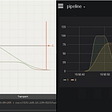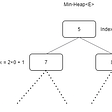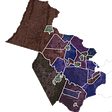# Daily Fantasy and Data Science: a Match Made in Degenerate Heaven

`df = pd.read_csv("FanDuel-NFL-2020-11-01-51232-players-list.csv")`
`df = df[df.columns[0:10]]`
`df_playable = df[(df.fppg > 1)&(df.played > 5)&(df.played < 8)]   `
`df_RB = df_playable[df_playable.position == 'RB']df_WR = df_playable[df_playable.position == 'WR']df_QB = df_playable[df_playable.position == 'QB']df_TE = df_playable[df_playable.position == 'TE']df_FLEX = df_playable[(df_playable.position == 'RB')|(df_playable.position == 'TE')|(df_playable.position == 'TE')]df_D = df_playable[df_playable.position == 'D']`
`lineup = []for x in range(1,10000):    RB = df_RB.sample(2)    WR = df_WR.sample(3)    FLEX = df_FLEX.sample(1)    QB = df_QB.sample(1)    DEF = df_D.sample(1)    TE = df_TE.sample(1)    df1 = RB.append(WR).append(FLEX).append(QB).append(DEF).append(TE)    lineup.append(df1)`
`for x in lineup:    x['salary_total'] = x.salary.sum()feasible_lineups = []for x in lineup:    if 60000 > x.salary_total.mean() > 50000:        feasible_lineups.append(x)def totalp(x):    return x.fppg.sum()feasible_lineups.sort(key = totalp, reverse = False)`
• QB: Russel Wilson, 28 pts
• RB: Rex Burkhead, 3 pts
• RB: Alvin Kamara: 20.8 pts
• WR: JuJu: 13pts
• WR: Zach Pascal: 7 pts
• WR: Tyler Locket: 7pts
• FLEX: Jimmy Graham: 3pts
• TE: Travis Kelce: 27pts
• D: LA Chargers: 2pts

--

--

--

## More from Aidan Coco

Love podcasts or audiobooks? Learn on the go with our new app.

## Airbnb Price Prediction: Model Selection & Results | Making Models (IV)## Real-time data processing pipeline showcase## 🍇 Better Batches with PyTorchText BucketIterator## Solving K-Max problem combining PriorityQueue and HashMap data structure## Data Structures and Algorithms Quiz## The Completion of my FIRST Case Study for Data Analysis## Data Analysis on FIFA international world cup data## Potentials of using social media to predict traffic accident## Floating Nirvana — Houseboats in India## Have you ever participated in a Stackoverflow survey?## Future of the Cybersecurity Job Market 2022## Want to Learn the Top 5 Skills Scientists Suggest for Successful Planet Heroes?